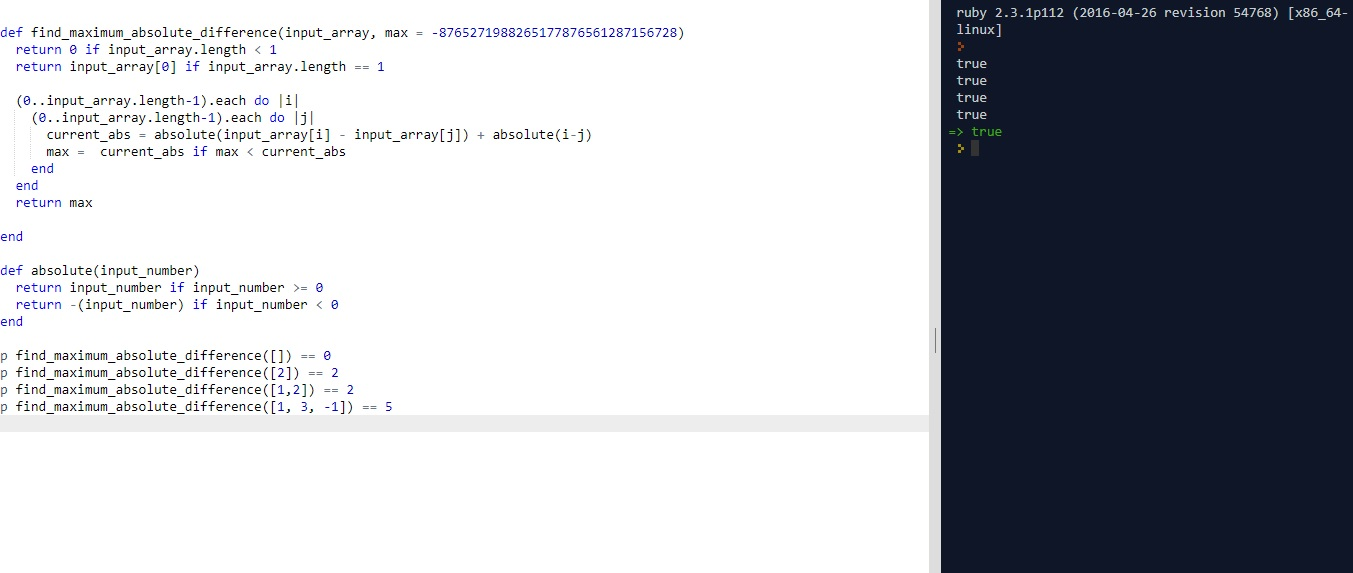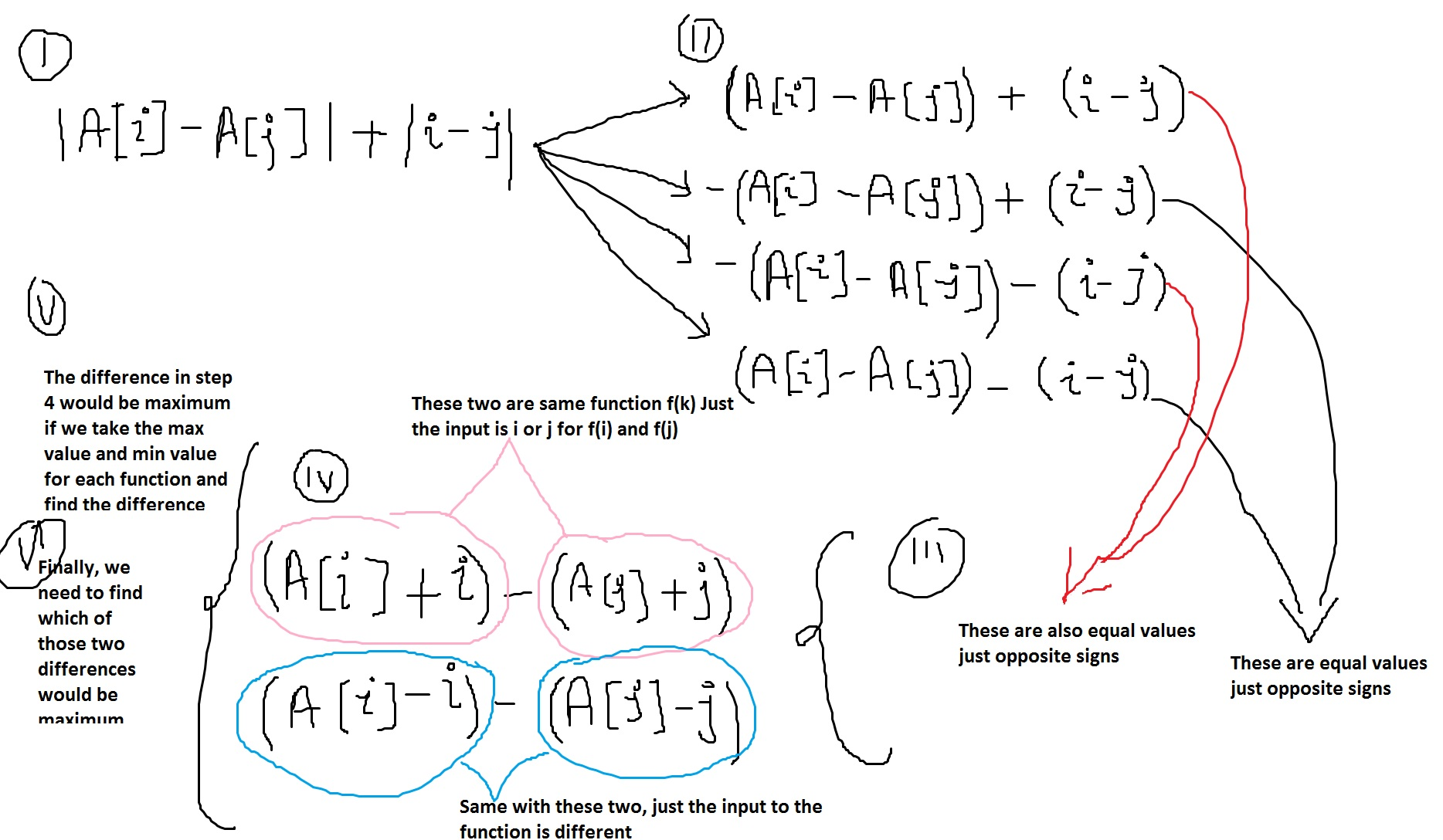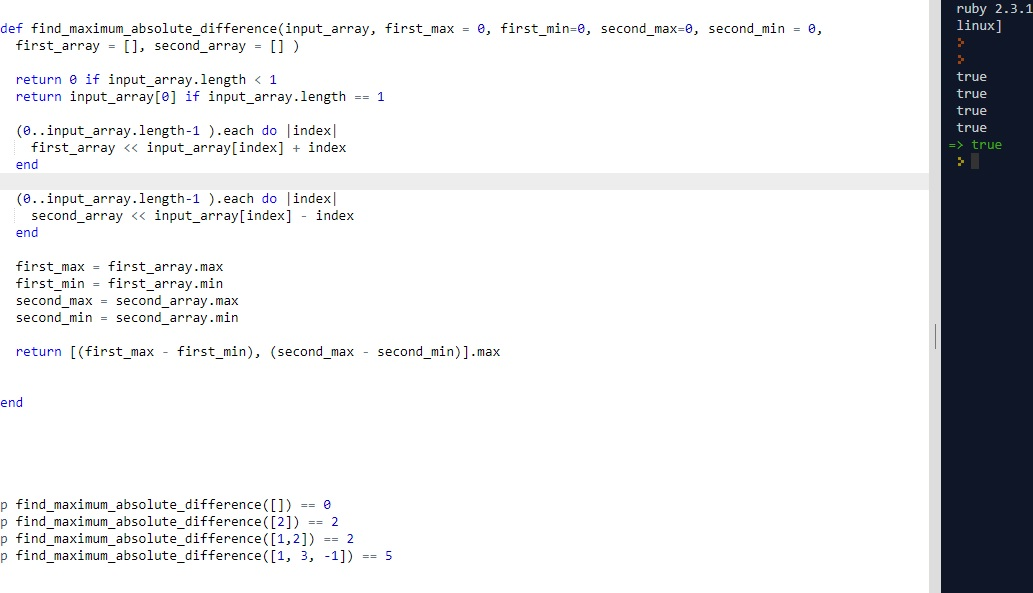# (*) CLEAN CODE: MAXIMUM ABSOLUTE DIFFERENCE

By: Saurav

2017-11-07 21:39:00 UTC

You are given an array of N integers, A1, A2 ,…, AN. Return maximum value of f(i, j) for all 1 ≤ i, j ≤ N.
f(i, j) is defined as |A[i] - A[j]| + |i - j|, where |x| denotes absolute value of x.

For example,

A=[1, 3, -1]

f(1, 1) = f(2, 2) = f(3, 3) = 0
f(1, 2) = f(2, 1) = |1 - 3| + |1 - 2| = 3
f(1, 3) = f(3, 1) = |1 - (-1)| + |1 - 3| = 4
f(2, 3) = f(3, 2) = |3 - (-1)| + |2 - 3| = 5

So, we return 5.

InterviewBit

Lets start by writing some tests first.

```p find_maximum_absolute_difference([]) == 0
p find_maximum_absolute_difference() == 2
p find_maximum_absolute_difference([1,2]) == 2
p find_maximum_absolute_difference([1, 3, -1]) == 5
```

Attempt 1
A very basic strategy would be to iterate through the array in nested loops and set value of i and j in the equation and update our max for a max value greater than what we already know.

```def find_maximum_absolute_difference(input_array, max = -8765271988265177876561287156728)
return 0 if input_array.length < 1
return input_array if input_array.length == 1

(0..input_array.length-1).each do |i|
(0..input_array.length-1).each do |j|
current_abs = absolute(input_array[i] - input_array[j]) + absolute(i-j)
max =  current_abs if max < current_abs
end
end
return max

end

def absolute(input_number)
return input_number if input_number >= 0
return -(input_number) if input_number < 0
end ```As we can see above, our tests pass and we got a solution.

But the big O time complexity is O(n^2) which tells me we gotta find another way.

Figure below explains the process in six stepsAttempt 2:

```def find_maximum_absolute_difference(input_array, first_max = 0, first_min=0, second_max=0, second_min = 0, first_array = [], second_array = [] )

return 0 if input_array.length < 1
return input_array if input_array.length == 1

(0..input_array.length-1 ).each do |index|
first_array << input_array[index] + index
end

(0..input_array.length-1 ).each do |index|
second_array << input_array[index] - index
end

first_max = first_array.max
first_min = first_array.min
second_max = second_array.max
second_min = second_array.min

return [(first_max - first_min), (second_max - second_min)].max

end ```As we see all our tests pass.

So, its time to refactor our code and make it a clean one following single responsibility and other clean code principles.

A refresher is right here

The Final Code:

```def find_maximum_absolute_difference(input_array)
return 0 if input_array.length < 1
return input_array if input_array.length == 1
first_array, second_array = build_first_function_array(input_array), build_second_function_array(input_array)
return [(first_array.max - first_array.min), (second_array.max - second_array.min)].max
end

def build_first_function_array(input_array, first_array = [])
(0..input_array.length-1 ).each do |index|
first_array << input_array[index] + index
end
return first_array
end

def build_second_function_array(input_array, second_array = [])
(0..input_array.length-1 ).each do |index|
second_array << input_array[index] - index
end
return second_array
end ```

Owned & Maintained by Saurav Prakash

If you like what you see, you can help me cover server costs or buy me a cup of coffee though donation :)